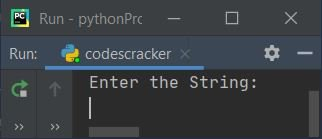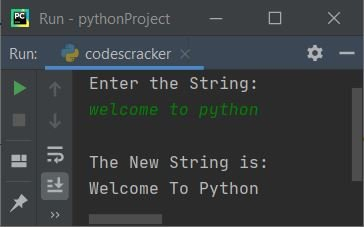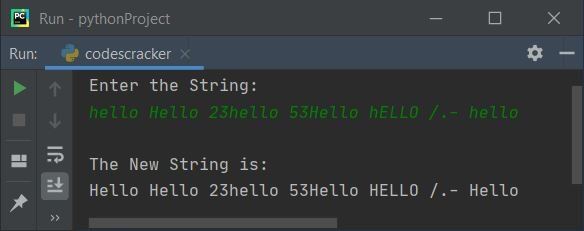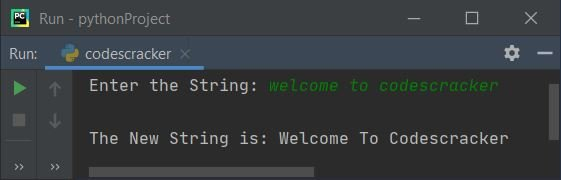# Python program to capitalize each word in a string

In this article, we've created some programs in Python to capitalize each word in a string entered by the user. Here is the list of programs:

• Capitalize the first letter of each word of the given string.
• Capitalize the first letter of each word using a list.
• Capitalize all words in a string using list and upper().
• Capitalize all words in a string using title().

## Capitalize the first letter of each word of the given string

This program capitalizes the first letter of each word of a string entered by the user. The question is: write a Python program to capitalize each word of a given string. Here is its answer:

```print("Enter the String: ")
text = str(input())
textLen = len(text)
for i in range(textLen):
ch = text[i]
if i==0:
ascVal = ord(ch)
if ascVal>=97 and ascVal<=122:
ascVal = ascVal-32
ascVal = chr(ascVal)
index = 0
text = text[:index] + ascVal + text[index+1:]
if ch==" ":
ch = text[i+1]
ascVal = ord(ch)
if ascVal>=97 and ascVal<=122:
ascVal = ascVal-32
ascVal = chr(ascVal)
index = i+1
text = text[:index] + ascVal + text[index+1:]
print("\nThe New String is:")
print(text)```

Here is its sample run:Now supply the string, say welcome to python and press the ENTER key to capitalize all three words of the given string, as shown in the snapshot given below:Note: The ord() function is used to convert a character to an integer (ASCII value). Because it returns the Unicode of a character passed as its argument.

Note: The chr() function is used to return the equivalent character of the Unicode value passed as its argument.

The dry run of the above program with user input, welcome to python goes like this:

• When the user enters the string, it gets stored in the text variable. So text="welcome to python"
• len(text) or len(welcome to python) or 17 gets initialized to textLen.
• Now the execution of the for loop begins. The range() method returns a sequence of values starting with 0 and incrementing by 1 by default. It stops before the value specified as its argument.
• At first execution, i=0 and the condition i<textLen or 0<17 evaluates to true; therefore, program flow goes inside the loop.
• text[i] or text or w gets initialized to ch.
• The condition (of if) i==0 or 0==0 evaluates to true, therefore program flow goes inside the if's body.
• And ord(ch) or ord(w) or 119 (Unicode of w) gets initialized to ascVal.
• Now the first condition (of the second if) ascVal>=97 or 119>=97 evaluates to true, and the second condition (of the second if) ascVal<=122 or 119<=122 evaluates to true. Since both conditions evaluates to true, program flow goes inside the second if's body.
• ascVal-32 or 119-32 or 87 (the ASCII value of capital W) gets initialized to ascVal.
• chr(ascVal) or chr(87) or W gets initialized to ascVal.
• 0 gets initialized to index. And the following statement
`text = text[:index] + ascVal + text[index+1:]`
states that the character of the indexth or 0th index of text gets capitalized.
• Now the condition (of another if) ch==" " gets evaluated, since ch's value is not equal to a space right now, therefore the condition evaluates to false, and the program flow goes back (to the for loop) and increments the value of i. That is, now i's value becomes 1 and checks whether it is less than textLen or not.
• Since the condition i<textLen or 1<17 evaluates to true again, program flow again goes inside the loop. This process continues until the condition is evaluates as false.
• In this way, each word of the given string gets capitalized.

In the above program, there are two ifs inside the loop. The first condition is applied to check and capitalize the first character of a string or the first character of a word. And the second if is applied to check for space and then operate with the next character from this space to check and capitalize.

## Capitalize the first letter of each word using a list

This program uses lists to do the same job as the previous program. The join() method joins or appends the thing.

```print("Enter the String: ")
text = str(input())
textLen = len(text)
textList = list(text)
for i in range(textLen):
ch = textList[i]
if i==0:
ascVal = ord(ch)
if ascVal>=97 and ascVal<=122:
ascVal = ascVal-32
ascVal = chr(ascVal)
index = 0
textList[index] = ascVal
text = "".join(textList)
if ch==" ":
ch = textList[i+1]
ascVal = ord(ch)
if ascVal>=97 and ascVal<=122:
ascVal = ascVal-32
ascVal = chr(ascVal)
index = i+1
textList[index] = ascVal
text = "".join(textList)
print("\nThe New String is:")
print(text)```

Here is a sample run with user input: hello Hello 23hello 53Hello hELLO /.- hello:## Capitalize all words in a string using List and upper()

This program uses the upper() function to capitalize all words of a given string at runtime.

```print(end="Enter the String: ")
text = str(input())
textLen = len(text)
textList = list(text)
for i in range(textLen):
ch = textList[i]
if i==0:
ascVal = ord(ch)
if ascVal>=97 and ascVal<=122:
index = 0
textList[index] = ch.upper()
text = "".join(textList)
if ch==" ":
ch = textList[i+1]
ascVal = ord(ch)
if ascVal>=97 and ascVal<=122:
index = i+1
textList[index] = ch.upper()
text = "".join(textList)
print("\nThe New String is: " + text)```

Here is its sample run with user input: welcome to codescracker:## Capitalize all words in a string using the title() function

This is the shortest program to capitalize all words of a given string using the title() function:

```print(end="Enter the String: ")
text = str(input())
text = text.title()
print("\nThe New String is: " + text)```

This program produces the same output as the previous program.

Python Online Test

« Previous Program Next Program »